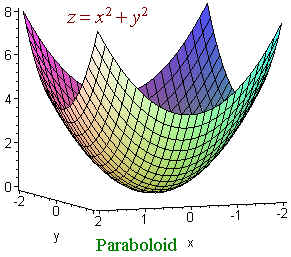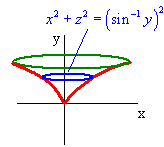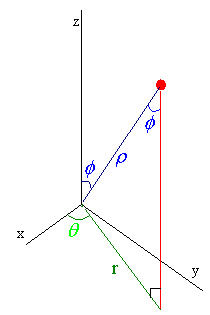$$\newcommand{\id}{\mathrm{id}}$$ $$\newcommand{\Span}{\mathrm{span}}$$ $$\newcommand{\kernel}{\mathrm{null}\,}$$ $$\newcommand{\range}{\mathrm{range}\,}$$ $$\newcommand{\RealPart}{\mathrm{Re}}$$ $$\newcommand{\ImaginaryPart}{\mathrm{Im}}$$ $$\newcommand{\Argument}{\mathrm{Arg}}$$ $$\newcommand{\norm}{\| #1 \|}$$ $$\newcommand{\inner}{\langle #1, #2 \rangle}$$ $$\newcommand{\Span}{\mathrm{span}}$$

# 1.8: Surfaces

$$\newcommand{\vecs}{\overset { \rightharpoonup} {\mathbf{#1}} }$$ $$\newcommand{\vecd}{\overset{-\!-\!\rightharpoonup}{\vphantom{a}\smash {#1}}}$$$$\newcommand{\id}{\mathrm{id}}$$ $$\newcommand{\Span}{\mathrm{span}}$$ $$\newcommand{\kernel}{\mathrm{null}\,}$$ $$\newcommand{\range}{\mathrm{range}\,}$$ $$\newcommand{\RealPart}{\mathrm{Re}}$$ $$\newcommand{\ImaginaryPart}{\mathrm{Im}}$$ $$\newcommand{\Argument}{\mathrm{Arg}}$$ $$\newcommand{\norm}{\| #1 \|}$$ $$\newcommand{\inner}{\langle #1, #2 \rangle}$$ $$\newcommand{\Span}{\mathrm{span}}$$ $$\newcommand{\id}{\mathrm{id}}$$ $$\newcommand{\Span}{\mathrm{span}}$$ $$\newcommand{\kernel}{\mathrm{null}\,}$$ $$\newcommand{\range}{\mathrm{range}\,}$$ $$\newcommand{\RealPart}{\mathrm{Re}}$$ $$\newcommand{\ImaginaryPart}{\mathrm{Im}}$$ $$\newcommand{\Argument}{\mathrm{Arg}}$$ $$\newcommand{\norm}{\| #1 \|}$$ $$\newcommand{\inner}{\langle #1, #2 \rangle}$$ $$\newcommand{\Span}{\mathrm{span}}$$

## Cylinders

Let C be a curve, then we define a cylinder to be the set of all lines through C and perpendicular to the plane that C lies in.We can tell that an equation is a cylinder is it is missing one of the variables.

Recall that the quadrics or conics are lines , hyperbolas, parabolas, circles, and ellipses. In three dimensions, we can combine any two of these and make a quadric surface. For exampleis a paraboloid since for constant z we get a circle and for constant x or y we get a parabola. We use the suffix -oid to mean ellipse or circle. We have:

\begin{align} \dfrac{x^2}{a^2} + \dfrac{y^2}{b^2 } + \dfrac{z^2}{c^2} &=1 \;\;\; \text{is an ellipsoid,} \\ -\dfrac{x^2}{a^2} - \dfrac{y^2}{b^2 } + \dfrac{z^2}{c^2} &=1 \;\;\; \text{is a hyperboloid of 2 sheets, while} \\ \dfrac{x^2}{a^2} + \dfrac{y^2}{b^2 } - \dfrac{z^2}{c^2} &=1 \;\;\; \text{is a hyperboloid of 1 sheet.} \end{align}

## Surface of Revolution

Let $$y = f(x)$$ be a curve, then the equation of the surface of revolution abut the x-axis is

$y^2 + z^2 = f^2(x).$Example $$\PageIndex{1}$$

Find the equation of the surface that is formed when the curve

$y = \sin x$

with

$0 \le x < \dfrac{\pi}{2}$

is revolved around the y-axis.

Solution

This uses a different formula since this time the curve is revolved around the y-axis.The circular cross section has radius $$\sin^{-1}y$$ and the circle is perpendicular to the y-axis. Hence the equation is

$x^2 + z^2 = (\sin^{-1} y)^2.$

## Cylindrical Coordinates

We can extend polar coordinates to three dimensions by

$x = r\, \cos\, q,$

$y = r\, \sin\, q,$

$z = z.$

Example $$\PageIndex{2}$$

We can write $$(1,1,3)$$ in cylindrical coordinates. We find

$r = \sqrt{1^2 + 1^2} = sqrt{2}$

and

$\theta = \tan^{-1} \left(\dfrac{1}{1} \right) = \dfrac{\pi}{4}$

so that the cylindrical coordinates are

$( \sqrt{2}, \frac{\pi}{4},3).$

## Spherical Coordinates

An alternate coordinate system works on a distance and two angle method called spherical coordinates. We let $$r$$ denote the distance from the point to the origin, $$\theta$$ represent the same $$\theta$$ as in cylindrical coordinates, and $$\phi$$ denote the angle from the positive z-axis to the point.The picture tells us that

$r = r \sin \phi$

and that

$z = r \cos \phi .$

From this we can find

• $$x = r\cos \theta = r \sin \phi \cos \theta$$,
• $$y = r \sin \theta = r \sin \phi \sin \theta$$,
• $$z = r \cos \phi$$.

Immediately we see that

$x^2 + y^2 + z^2 = r^2.$

We use spherical coordinates whenever the problem involves a distance from a source.

Example $$\PageIndex{3}$$

Convert the surface

$z = x^2 + y^2$

to an equation in spherical coordinates.

Solution

We add $$z^2$$ to both sides

$z + z^2 = x^2 + y^2 + z^2.$

Now it is easier to convert

$r\, \cos \phi + r^2 \, \cos^2 \phi = r^2.$

Divide by $$r$$ to get

$\cos \phi + r\, \cos^2 \phi = r .$

Now solve for $$r$$.

$r = \dfrac{\cos \phi }{1 - \cos^2 \phi } = \dfrac{\cos \phi }{\sin^2 \phi } = \csc \phi \; \cot \phi .$

Larry Green (Lake Tahoe Community College)

• Integrated by Justin Marshall.